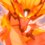# Closed Form of Generalized Trigonometric Products

$\text{a)}$ Find a closed form for the following Trigonometric Products

$\bullet \prod_{r=1}^{n-1} \sin \left(k \cdot \dfrac{r\pi}{n} \right)$

$\bullet \prod_{r=1}^{n-1} \cos \left(k \cdot \dfrac{r\pi}{n}\right)$

Here $k > 1$ is a positive integer.

$\text{b)}$ Generalize for all complex $k$

This is a part of the set Formidable Series and Integrals.Note by Ishan Singh
3 years, 9 months ago

This discussion board is a place to discuss our Daily Challenges and the math and science related to those challenges. Explanations are more than just a solution — they should explain the steps and thinking strategies that you used to obtain the solution. Comments should further the discussion of math and science.

When posting on Brilliant:

• Use the emojis to react to an explanation, whether you're congratulating a job well done , or just really confused .
• Ask specific questions about the challenge or the steps in somebody's explanation. Well-posed questions can add a lot to the discussion, but posting "I don't understand!" doesn't help anyone.
• Try to contribute something new to the discussion, whether it is an extension, generalization or other idea related to the challenge.

MarkdownAppears as
*italics* or _italics_ italics
**bold** or __bold__ bold
- bulleted- list
• bulleted
• list
1. numbered2. list
1. numbered
2. list
Note: you must add a full line of space before and after lists for them to show up correctly
paragraph 1paragraph 2

paragraph 1

paragraph 2

[example link](https://brilliant.org)example link
> This is a quote
This is a quote
    # I indented these lines
# 4 spaces, and now they show
# up as a code block.

print "hello world"
# I indented these lines
# 4 spaces, and now they show
# up as a code block.

print "hello world"
MathAppears as
Remember to wrap math in $$ ... $$ or $ ... $ to ensure proper formatting.
2 \times 3 $2 \times 3$
2^{34} $2^{34}$
a_{i-1} $a_{i-1}$
\frac{2}{3} $\frac{2}{3}$
\sqrt{2} $\sqrt{2}$
\sum_{i=1}^3 $\sum_{i=1}^3$
\sin \theta $\sin \theta$
\boxed{123} $\boxed{123}$

Sort by:

@Otto Bretscher @Mark Hennings What are your thoughts on this?

- 3 years, 9 months ago

I'm at work now; just a quick reply. Those products are not hard to do with complex numbers. The sine product, obviously, is 0 when $\gcd(k,n)\neq 1$, and it is $\frac{n}{2^{n-1}}$ in absolute value when $\gcd(n,k)=1$... the roots of unity get permuted in that case. For the cosine it gets just a bit messier but it is also very doable.

You can play with my solution here to get those results.

- 3 years, 9 months ago

Okay. Sorry to disturb and thanks for the input. Btw, I had tried using roots of unity for cosine but was getting a contradiction (that was a year ago, I'll have to re-check my work now).

- 3 years, 9 months ago

Try it yourself! If you use the complex expressions for sin and cosine, the computations are quite straightforward, and fun.

- 3 years, 9 months ago

I'll try it again, maybe I did some calculation mistake when I last tried it a year ago.

- 3 years, 9 months ago

We know that roots of unity work for the cosine when $k=1$... look at my solution here. It works the exact same way when $\gcd(n,k)=1$. Now tackle the case $\gcd(n,k)\neq 1$.

- 3 years, 9 months ago

Hmm. I found my flaw. It's pretty straight forward with roots of unity. I was earlier committing a calculation mistake and getting a contradiction when I tried using roots of unity. I also tried using Chebyshev Polynomials on the lines of my solution here but it got somewhat messy.

- 3 years, 9 months ago

I love Chebyshev polynomials, but here roots of unity seem like the way to go.

Are we done with this, or do you want to pursue it further?

- 3 years, 9 months ago

- 3 years, 9 months ago

I have solved it for both cases. I'll post solution by night.

- 3 years, 9 months ago

Finding the signs is a straightforward but tedious book keeping issue; let's just go for the absolute values for now since I have limited time.

In the sign product, the $r$ should go from 1 to $n-1$; the way it's written now the product is always 0 ;)

I will essentially be copying my solutions from here and here

If $\gcd(k,n)\neq 1$ then the product is 0 as $kr$ will be a multiple of $n$ for some $r$.

If $\gcd(k,n)=1$ then

$\prod_{r=1}^{n-1}2|\sin(kr\pi/n)|=\prod_{r=1}^{n-1}|e^{ikr\pi/n}-e^{-ikr\pi/n}|=\prod_{r=1}^{n-1}|1-e^{2ikr\pi/n}|=n$

so that $\prod_{r=1}^{n-1}|\sin(kr\pi/n)|=\frac{n}{2^{n-1}}$

Likewise, if $\gcd(k,n)=1$ and $n$ is odd, then

$\prod_{r=1}^{n}2|\cos(kr\pi/n)|=\prod_{r=1}^{n}|e^{ikr\pi/n}+e^{-ikr\pi/n}|=\prod_{r=1}^{n}|-1-e^{2ikr\pi/n}|=2$

so that $\prod_{r=1}^{n}|\cos(kr\pi/n)|=\frac{1}{2^{n-1}}$

If $k=pq$ where $p=\gcd(k,n)$, let $n=mp$. Then

$\prod_{r=1}^{n}|\cos(kr\pi/n)|=\prod_{r=1}^{pm}|\cos(qr\pi/m)|=\left(\frac{1}{2^{m-1}}\right)^p=\frac{1}{2^{n-\gcd(n,k)}}$

- 3 years, 9 months ago

@Otto Bretscher Sorry to disturb again, but when I inspected my earlier work, I found that I was trying to generalize the result for all complex $k$. I tried to use transformation of roots on the $n^{th}$ roots of unity, but to no avail. Can this be done?

- 3 years, 9 months ago

It's a nice sunny spring day here, and I don't feel like thinking about messy stuff like this right now. Maybe on a rainy day... ;)

- 3 years, 9 months ago

Yeah sure :) Thanks for the help.

- 3 years, 9 months ago

(+1) Nice solution! My solution is essentially the same as yours, except instead of using cyclotomic polynomials, if $\gcd (k,n) =1$ , note that $\displaystyle \prod_{r=1}^{n-1} \sin \left(\dfrac{rk \pi}{n} \right) = (-1)^{n-1} \prod_{r=1}^{n-1} \sin \left(\dfrac{r \pi}{n} \right) = (-1)^{n-1} \dfrac{n}{2^{n-1}}$ since the set $rk \pmod {n}$ is exhaustive for $1 \leq r \leq n-1$. Similarly for $\cos$

- 3 years, 9 months ago

Yes, of course, I wasn't using the cyclotomic polynomials in this problem either. I was using them for the other problem because there we were restricting ourselves to the $r$ with $\gcd(r,n)=1$.

- 3 years, 9 months ago

No problem at all. I will write the solutions up in the evening unless somebody else does the work ;)

- 3 years, 9 months ago